## Embedding GeoGebra Apps into SLS

Teachers in Singapore have been provided with the Student Learning Space (SLS) - an online platform meant for students' self-directed learning using MOE's curriculum-aligned resources as well as for teachers to create engaging technology-assisted learning experiences for their charges.

One of the features that many science and math teachers find useful is the ability to create links out to simulations and other interactive apps. Those who know how can also embed html5 packages into their "Lesson" so as to provide a more seamless experience for their students. These packages must be stand-alone packages that do not require external sources such as sound files or images. In other words, every media file that is needed for the html5 package to run must be all zipped up into a folder before uploading.

Here, I will go through step-by-step how to embed a GeoGebra app into SLS.

Step 1: Find a suitable GeoGebra App (https://www.geogebra.org)

You might like to check out the list of GeoGebra apps that I've curated for Singapore's O-level and A-level syllabi.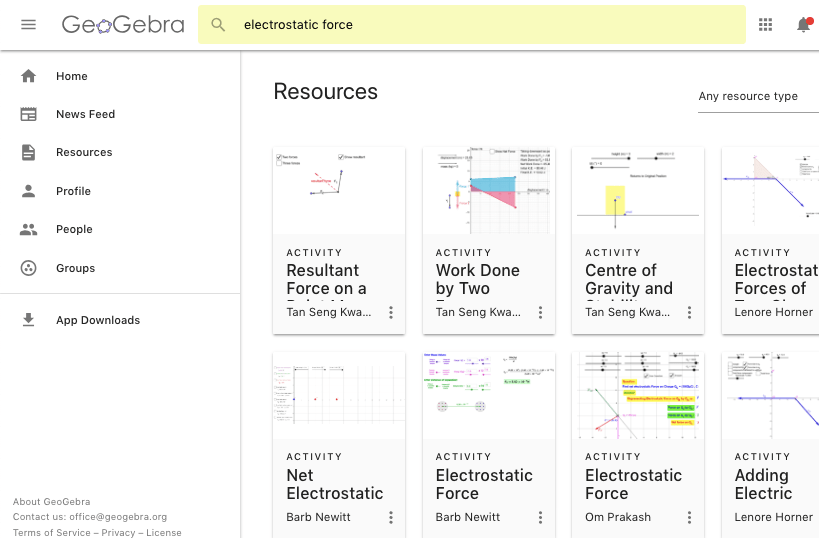Step 2: Open the App and click to see Details.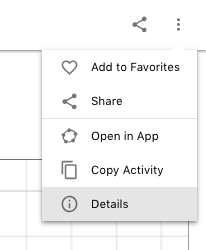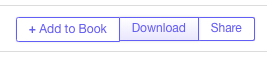Step 4: Unzip the package and rename the html file to index.html.

What the file name looks like before: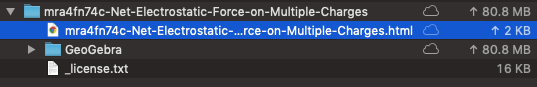What the file name should look like after:Step 4: Zip up the files again. Select all the files including the index.html file and zip it up. Do not zip the main folder. Select only the index.html and the folder with the title GeoGebra. The index.html file must be in the root directory of the new zip folder.The new zip folder could be given any name.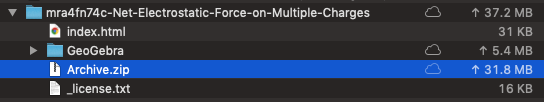Step 5: In SLS, select the Lesson and Activity in which you want your GeoGebra app to appear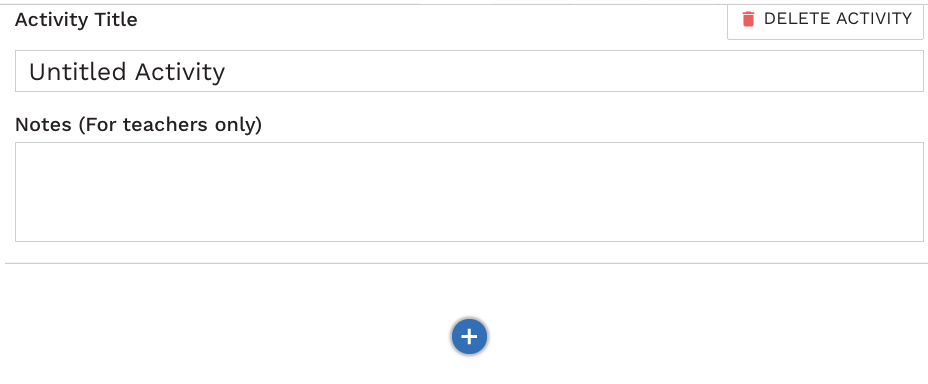Step 6: Select a new "Media" objectStep 7: Click UploadStep 8: Browse to find the zip folder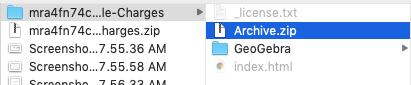You should see this message. That is a sign that your package is correctly packaged.Finally, you can try out the app in the student view.If you need any assistance, feel free to leave a comment below. I will try my best to support you. In the worst case scenario, I can create the SLS lesson with the desired GeoGebra app and share it with you.

NOTE (ON 2 JUL) : With the new SLS embed function, you can also choose to embed the live GeoGebra App using an iframe following the method found here.

## Geogebra App on Maximum Power Theorem

This simulation demonstrates the power dissipated in a variable resistor given that the battery has an internal resistance (made variable in this app as well).

Since the power dissipated by the resistor is given by

$P=I^2R$

and the current is given by

$I=E(R+r)$,

$P= E^2\times\frac{R}{(R+r)^2}=\frac{E^2}{\frac{r^2}{R}+R+2r}$

This power will be a maximum if the expression for the denominator

$\frac{r^2}{R}+R+2r$

is a minimum.

Differentiating the expression with respect to R, we get

$\frac{d(r^2/R+R+2r)}{dR}=-\frac{r^2}{R^2}+1$

When the denominator is a minimum,

$-\frac{r^2}{R^2}+1=0$, so

r = R.

## Geogebra Simulation of a Potentiometer

Some of the more challenging problems in the topic of electricity in the A-level syllabus are those involving a potentiometer. The solution involves the concept of potential divider and the setup can be used to measure emf or potential difference across a variety of circuits components. Basically, students need to understand the rule - that the potential difference across a device is simply a fraction of the circuit's emf, and that fraction is equal to the resistance of the device over the total resistance of the circuit.

$V_{device}=\frac{R_{device}}{R_{total}}*emf$

The intention of this Geogebra app is for students to practise working on their calculations, as well as to reinforce their understanding of the principle by which the potentiometer works.

## Geogebra Apps for Singapore Physics

I've curated a series of Geogebra apps that are relevant and useful for the instructional objectives under the Singapore-Cambridge GCE 'O' and 'A' level syllabi. Some of these apps were created by myself. If you have any ideas for new Geogebra apps, do let me know in the comments section below and I'll see if it's possible to create. Alternatively, if you have come across other Geogebra apps that can be relevant to the local physics syllabus, I would gladly include them into my lists!

## Box on a Vertical Oscillating Spring - Geogebra App

Students can explore how varying frequency and amplitude of the vertical oscillation of a platform could cause an object resting on it to temporarily leave the platform (i.e. when normal contact force is zero).

## PV Diagram for an Ideal Gas

Applying the 1st Law of Thermodynamics to 4 simple changes on an ideal gas, students can check their understanding using this Geogebra app. When is work done positive? Which processes bring about an increase in internal energy or temperature? Which processes require heat input?

## Geogebra App for Kinematics

As one of the first topics in A-level physics, kinematics introduces JC students to the variation of velocity and displacement with acceleration. Very often, they struggle with the graphical representations of the 3 variables.

This Geogebra app allows students to vary acceleration (keeping it to a linear function for simplicity) while observing changes to velocity and displacement. Students can also change the initial conditions of velocity and displacement.

The default setting shows an object being thrown upwards with downward gravitational acceleration of 10 m s-2.

The movement of the particle with time is shown on the left with a reference line showing the position on the displacement graph.

## Wavefront

In order to help students visualise a wavefront, a 3-D image is usually used to show the imaginary line joining particles in phase. I created the Geogebra apps below to allow students to change the wavefront and observe it move with time at a constant wave speed. There represent simplified versions of waves on a ripple tank with a linear and circular wavefront.